GRE Subject Test: Chemistry : Reaction Equilibrium

Example Questions

Example Question #7 : Equilibrium Constant And Reaction Quotient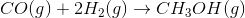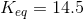The initial concentrations of this reaction are listed below.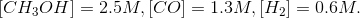Based on these initial concentrations, which statement is true?

The forward rate will be greater than the reverse rate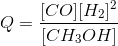The reaction is in equilibrium

The ratio of products to reactants is greater than the equilibrium constant

The reaction will shift left

The forward rate will be greater than the reverse rate

Explanation:

When given initial concentrations, we can determine the reaction quotient (Q) of the reaction. The reaction quotient is given by the same equation as the equilibrium constant (concentration of products divided by concentration of reactants), but its value will fluctuate as the system reacts, whereas the equilibrium constant is based on equilibrium concentrations.

By comparing the reaction quotient to the equilibrium constant, we can determine in which direction the reaction will proceed initially.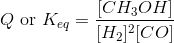The reaction quotient with the beginning concentrations is written below.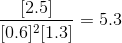This shows that the ratio of products to reactants is less than the equilibrium constant. Since Q is less than Keq in the beginning, we conclude that the reaction will proceed forward until Q is equal to Keq. In this manner, the denominator (reactants) will decrease and the numerator (products) will increase, causing Q to become closer to Keq.

Example Question #8 : Le Chatelier's Principle And Common Ion Effect

Consider the reaction reaction below.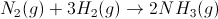A student allows the system to reach equilibrium and then removes two moles of hydrogen gas. Which of the following will be a result?

The reaction will shift to the side with fewer total moles of gas

More NH3 will be produced

The reaction will first shift toward the products, then toward the reactants

No change will occur

The amount of N2 in the reaction vessel will increase

The amount of N2 in the reaction vessel will increase

Explanation:

According to Le Chatelier's principle, when a system at equilibrium is disturbed, the system will react to restore equilibrium. In other words, it will seek to undo the stress. Here, if hydrogen gas is removed, the reaction will shift toward the reactants to re-form it. In the process, more nitrogen will be produced.

Example Question #161 : General Chemistry

A saturated solution of scandium hydroxide, which also contains solid scandium hydroxide, is treated with 0.1N HCl. The addition of acid will __________ the solubility of scandium hydroxide because of __________.

increase . . . Le Chatelier's principle

decrease . . . the common ion effect

increase . . . the decreased pH

not affect . . . the common ion effect

decrease . . . Le Chatelier's principle

increase . . . Le Chatelier's principle

Explanation:

Scandium hydroxide dissociates according to this reaction.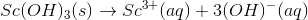As 0.1N HCl is added, it will quantitatively react with hydroxide anions to produce ScCl3 and water.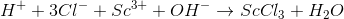Hydroxide will be removed from the above equilibrium, and the system will compensate by shifting the equilibrium to the right, according Le Chatelier's principle. As the equilibrium shifts to the right, more solid scandium hydroxide is hydrolyzed, resulting in increased solubility.

Example Question #162 : General Chemistry

A system contains iodine atoms that are in equilibrium with respect to the reaction below: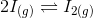The volume of the system is suddenly reduced, leading to an increase in pressure. What effect will this have on the reaction?

The reaction shifts to the right then to the left

The reaction will not be affected

The reaction shifts to the left

The reaction shifts to the right

The reaction shifts to the right

Explanation:

Since the system is originally at equilibrium and a stress is applied, Le Chatelier's principle is to be considered. By increasing the total pressure, the reaction will move in the direction toward which there are less molecules of gas.Looking at the original reaction there are two moles of gas on the left and only one on the right, indicating that the reaction will shift to the right if the pressure is increased.

Example Question #1 : Reaction Equilibrium

Consider the following saturated solution. Assume it is at equilibrium.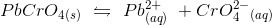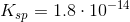What is the chromate ion concentration after 0.019 moles of lead nitrate is added to 1 L of the above solution?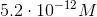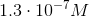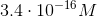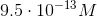Explanation:

Us the solubility constant to calculate the answer to this question. We know that: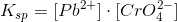andSince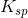is a very small number, we can assume that the concentration of chromate,, will be very small compared to the concentration of the added lead, 0.019 M. We can use the two known values (and lead concentration) to solve for the unknown chromate concentration: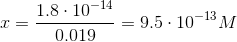Example Question #161 : General Chemistry

Which method can be used to increase the activation energy of a reaction done in the lab?

Shaking the reaction flask

Decreasing the temperature

Increasing the temperature

Increasing the concentration of reactants

Increasing the pressure

Decreasing the temperature

Explanation:

The activation energy is the minimum amount of energy required to start a chemical reaction. Based on the collision model, increasing the temperature, pressure, and concentration for a chemical reaction will decrease the activation energy. This is because increasing these factors increases the number of collisions of molecules, which is required for a reaction to occur. Therefore, decreasing the temperature would have the opposite effect causing the activation energy to increase, decreasing the likelihood of a reaction taking place.

Example Question #1 : Reaction Equilibrium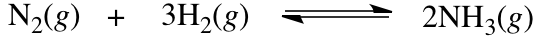Based on the equilibrium reaction shown, how would adding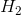to the system affect the equilibrium?

Addingto the system would cause the equilibrium to shift to the right, producing more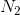in order to reduce the concentration of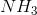The effect of addingto the system cannot be predicted

Addingto the system would cause the equilibrium to shift to the left, producing morein order to reduce the concentration ofAddingwould not have any effect on the equilibrium

Addingto the system would cause the equilibrium to shift to the right, producing morein order to reduce the concentration ofAddingto the system would cause the equilibrium to shift to the right, producing morein order to reduce the concentration ofExplanation:

According to Le Chatelier's principle, if an equilibrium reaction is disturbed by addition of a reactant or product, the equilibrium will shift to counteract the disturbance. Therefore, addingto the system would cause the equilibrium to shift to the right, producing morein order to reduce the concentration of.

Example Question #162 : General Chemistry

Which method can be used to decrease the activation energy of a reaction?

Increasing the pressure

Increasing the concentration of reactants

All of these

Increasing the temperature